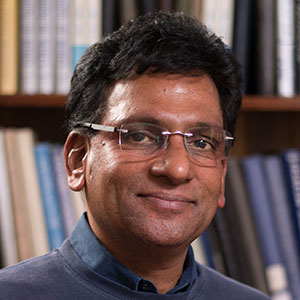Select Page

###### Professor, Applied Mathematics and Statistics Professor, Computer Science Affiliate Professor, Electrical Engineering### Contact

Chauvenet Hall 222
303-273-3927
Fax: 303-273-3875
mganesh@mines.edu

### Research

• Stochastic, model reduction and multiscle algorithms
• Quantification of uncertainties in parallel scientific computing models
• Computational electromagnetics & High-performance computing
• High-order, high-performance computing algorithms for wave propagation and absorption simulations in 3-D.
• Forward and inverse acoustic and electromagnetic scattering in 3-D.
• Efficient algorithms for the Stokes and Navier-Stokes equations on rotating surfaces.
• Quadrature finite element methods for elliptic, parabolic and hyperbolic problems.
• Free surface nonlinear evolutionary systems with applications.
• Constructive approximations on spherical surfaces.
• Fully discrete spectral boundary integral and boundary element methods.
• Parallel evolutionary computations and analysis.
• Radial basis functions based numerical schemes for PDEs.

### Publications

• A fully discrete Galerkin method for high frequency exterior acoustic scattering in three dimensions .
(With S. C. Hawkins .) J. Comp. Phys. , 230 (2011), 104-125.
• An efficient algorithm for simulating scattering by a large number of two dimensional particles.
(With S. C. Hawkins .) Aust. & NZ Indus. Appl. Math. (ANZIAM) J., 52 (2011), C139-C155.
• A pseudospectral quadrature method for Navier-Stokes equations on rotating spheres
(With Q. T. Le Gia and I. H. Sloan .) Math. Comp. , 80 (2011), 1397-1430.
• A radial basis Galerkin method for spherical surface Stokes equations.
(With Q.T. Le Gia .) Aust. & NZ Indus. Appl. Math. (ANZIAM) J., 52 (2011), C58-C71.
• Convergence analysis with parameter estimates for a reduced basis acoustics scattering T-matrix method
(With S. C. Hawkins and R. Hiptmair .) IMA J. Numer. Anal., 32 (2012), 1348-1374.
• A reduced basis method for electromagnetic scatttering by multiple particles in three dimensions
(With J. Hesthaven and B. Stamm .) J. Comput. Phys., 231 (2012), 7756-7779
• A stochastic pseudospectral and T-matrix algorithm for acoustic scattering by a class of multiple particle configurations
(With S. C. Hawkins .) J. Quant. Spect. Radiative Transfer, 123 (2013), 41-52
• Interpolation and cubature approximation and analysis for a class of wideband integrals on the sphere
(With V. Dominguez .) Advances in Comput. Math., 39, 547-584, 2013.
• An efficient algorithm for simulation of stochastic scattering cross-sections
(With S. C. Hawkins .) Aust. & NZ Indus. Appl. Math. (ANZIAM) J., 54 (2013), C119-C136.
• An all-frequency weakly-singular surface integral equation for electromagnetism in dielectric media: Reformulation and well-posedness analysis
(With S. C. Hawkins and D. Volkov .) J. Math. Anal. & Appl., 412 (2014), 277-300.
• Spectral properties of Schrodinger operators on superconducting surfaces
(With T. Thompson .) J. Spectral Theory, 4 (2014), 569-612.
• An efficient O(N) algorithm for computing O(N^2) acoustic wave interactions in large N-obstacle three dimensional configurations
(With S. C. Hawkins .) BIT Numer. Math., 55(2015), 117-139.
• Schrodinger eigenbasis on a class of superconducting surfaces : Ansatz, analysis, FEM approximations and computations
(With T. Thompson .) Appl. Numer. Math., 89 (2015), 45-75.
• A high performance computing and sensitivity analysis algorithm for stochastic many-particle wave scattering
(With S. C. Hawkins .) SIAM J Scientific Computing, 37 (2015), A1475-A1503
• Sobolev estimates for constructive uniform-grid FFT interpolatory approximations of spherical functions
(With V. Dominguez .) Advances in Comput. Math., 42 (2016), 843-887.
• Scattering by stochastic boundaries: hybrid low- and high-order quantification algorithms
(With S. C. Hawkins .) Aust. & NZ Indus. Appl. Math. (ANZIAM) J., 56 (2016) C312-C338.
• High-order FEM-BEM computer models for wave propagation in unbounded and heterogeneous media: Application to time-harmonic acoustic horn problem
(With C. Morgenstern .) J. Comp. Appl. Math., 307 (2016) 183-203.
• An offline/online algorithm for a class of stochastic multiple obstacle configurations in half-plane.
(With S. C. Hawkins .) ) J. Comp. Appl. Math., 307 (2016) 52-64.
• Parallel mixed FEM simulation of a class of single-phase models with non-local operators
(With A. Alyoubi .) J. Comp. Appl. Math., 307 (2016)106-118.
• An efficient multigrid algorithm for heterogeneous acoustic media sign-indefinite high-order FEM model
(With C. Morgenstern .) Numer. Linear Algebra, 2017 (to appear, DOI: 10.1002/nla.2049).
• Computing spatial correlation of ground motion intensities for shakeMap
(With S. A. Verros, D. J. Wald, C. B. Worden, M. Hearne .) Computers & Geosciences, 37:106-118, 2017).
• TMATROM: A T-matrix Reduced Order Model Software
(With S. C. Hawkins .) ACM Trans. Math. Software, 2017 (to appear).
• Sign-definite preconditioned high-order FEM. Part 1: Formulation and simulation for acoustic wave propagation in bounded homogeneous media.
(With C. Morgenstern .) SIAM J Scientific Computing, 2017 (to appear).
• A spectrally accurate algorithm and analysis for a Ginzburg-Landau model on superconducting surfaces
(With T. Thompson .) SIAM J. Multiscale Model. Simul., 2017 (to appear).
• High-order FEM domain decomposition models for high-frequency wave propagation in heterogeneous media.
(With C. Morgenstern S .) Submitted.
• An FEM-MLMC algorithm for a moving shutter diffraction in time stochastic model.
(With B. Reyes and A. Purkayastha .) Submitted.

### Recent Courses

• MATH 440/540 Parallel Scientific Computing.
• BELS/MATH 433 Mathematical Biology.
• MATH 550 Numerical Methods for Partial Differential Equations.
• MATH 332/342 Linear Algebra.
• MATH 307 Introduction to Scientific Computing.